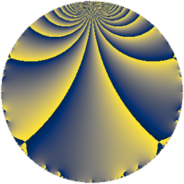# Properties

 Label 2280.2.a.oLevel $2280$ Weight $2$ Character orbit 2280.a Self dual yes Analytic conductor $18.206$ Analytic rank $1$ Dimension $2$ CM no Inner twists $1$

# Related objects

## Newspace parameters

 Level: $$N$$ $$=$$ $$2280 = 2^{3} \cdot 3 \cdot 5 \cdot 19$$ Weight: $$k$$ $$=$$ $$2$$ Character orbit: $$[\chi]$$ $$=$$ 2280.a (trivial)

## Newform invariants

 Self dual: yes Analytic conductor: $$18.2058916609$$ Analytic rank: $$1$$ Dimension: $$2$$ Coefficient field: $$\Q(\sqrt{2})$$ Defining polynomial: $$x^{2} - 2$$ Coefficient ring: $$\Z[a_1, \ldots, a_{7}]$$ Coefficient ring index: $$1$$ Twist minimal: yes Fricke sign: $$1$$ Sato-Tate group: $\mathrm{SU}(2)$

## $q$-expansion

Coefficients of the $$q$$-expansion are expressed in terms of $$\beta = \sqrt{2}$$. We also show the integral $$q$$-expansion of the trace form.

 $$f(q)$$ $$=$$ $$q + q^{3} - q^{5} + ( -2 + \beta ) q^{7} + q^{9} +O(q^{10})$$ $$q + q^{3} - q^{5} + ( -2 + \beta ) q^{7} + q^{9} + \beta q^{11} -3 \beta q^{13} - q^{15} -2 \beta q^{17} - q^{19} + ( -2 + \beta ) q^{21} + ( -2 + 2 \beta ) q^{23} + q^{25} + q^{27} + ( -2 - 3 \beta ) q^{29} + ( -6 + 2 \beta ) q^{31} + \beta q^{33} + ( 2 - \beta ) q^{35} + 5 \beta q^{37} -3 \beta q^{39} + ( -2 - 3 \beta ) q^{41} + ( -6 - 3 \beta ) q^{43} - q^{45} + ( 2 - 2 \beta ) q^{47} + ( -1 - 4 \beta ) q^{49} -2 \beta q^{51} + ( -4 + 6 \beta ) q^{53} -\beta q^{55} - q^{57} + 2 \beta q^{59} -8 q^{61} + ( -2 + \beta ) q^{63} + 3 \beta q^{65} + 8 \beta q^{67} + ( -2 + 2 \beta ) q^{69} + ( -8 - 2 \beta ) q^{71} + ( 2 + 4 \beta ) q^{73} + q^{75} + ( 2 - 2 \beta ) q^{77} + ( -8 - 4 \beta ) q^{79} + q^{81} -6 q^{83} + 2 \beta q^{85} + ( -2 - 3 \beta ) q^{87} + ( 2 + 11 \beta ) q^{89} + ( -6 + 6 \beta ) q^{91} + ( -6 + 2 \beta ) q^{93} + q^{95} -7 \beta q^{97} + \beta q^{99} +O(q^{100})$$ $$\operatorname{Tr}(f)(q)$$ $$=$$ $$2 q + 2 q^{3} - 2 q^{5} - 4 q^{7} + 2 q^{9} + O(q^{10})$$ $$2 q + 2 q^{3} - 2 q^{5} - 4 q^{7} + 2 q^{9} - 2 q^{15} - 2 q^{19} - 4 q^{21} - 4 q^{23} + 2 q^{25} + 2 q^{27} - 4 q^{29} - 12 q^{31} + 4 q^{35} - 4 q^{41} - 12 q^{43} - 2 q^{45} + 4 q^{47} - 2 q^{49} - 8 q^{53} - 2 q^{57} - 16 q^{61} - 4 q^{63} - 4 q^{69} - 16 q^{71} + 4 q^{73} + 2 q^{75} + 4 q^{77} - 16 q^{79} + 2 q^{81} - 12 q^{83} - 4 q^{87} + 4 q^{89} - 12 q^{91} - 12 q^{93} + 2 q^{95} + O(q^{100})$$

## Embeddings

For each embedding $$\iota_m$$ of the coefficient field, the values $$\iota_m(a_n)$$ are shown below.

For more information on an embedded modular form you can click on its label.

Label $$\iota_m(\nu)$$ $$a_{2}$$ $$a_{3}$$ $$a_{4}$$ $$a_{5}$$ $$a_{6}$$ $$a_{7}$$ $$a_{8}$$ $$a_{9}$$ $$a_{10}$$
1.1
 −1.41421 1.41421
0 1.00000 0 −1.00000 0 −3.41421 0 1.00000 0
1.2 0 1.00000 0 −1.00000 0 −0.585786 0 1.00000 0
 $$n$$: e.g. 2-40 or 990-1000 Significant digits: Format: Complex embeddings Normalized embeddings Satake parameters Satake angles

## Atkin-Lehner signs

$$p$$ Sign
$$2$$ $$-1$$
$$3$$ $$-1$$
$$5$$ $$1$$
$$19$$ $$1$$

## Inner twists

This newform does not admit any (nontrivial) inner twists.

## Twists

By twisting character orbit
Char Parity Ord Mult Type Twist Min Dim
1.a even 1 1 trivial 2280.2.a.o 2
3.b odd 2 1 6840.2.a.x 2
4.b odd 2 1 4560.2.a.bg 2

By twisted newform orbit
Twist Min Dim Char Parity Ord Mult Type
2280.2.a.o 2 1.a even 1 1 trivial
4560.2.a.bg 2 4.b odd 2 1
6840.2.a.x 2 3.b odd 2 1

## Hecke kernels

This newform subspace can be constructed as the intersection of the kernels of the following linear operators acting on $$S_{2}^{\mathrm{new}}(\Gamma_0(2280))$$:

 $$T_{7}^{2} + 4 T_{7} + 2$$ $$T_{11}^{2} - 2$$ $$T_{13}^{2} - 18$$

## Hecke characteristic polynomials

$p$ $F_p(T)$
$2$ $$T^{2}$$
$3$ $$( -1 + T )^{2}$$
$5$ $$( 1 + T )^{2}$$
$7$ $$2 + 4 T + T^{2}$$
$11$ $$-2 + T^{2}$$
$13$ $$-18 + T^{2}$$
$17$ $$-8 + T^{2}$$
$19$ $$( 1 + T )^{2}$$
$23$ $$-4 + 4 T + T^{2}$$
$29$ $$-14 + 4 T + T^{2}$$
$31$ $$28 + 12 T + T^{2}$$
$37$ $$-50 + T^{2}$$
$41$ $$-14 + 4 T + T^{2}$$
$43$ $$18 + 12 T + T^{2}$$
$47$ $$-4 - 4 T + T^{2}$$
$53$ $$-56 + 8 T + T^{2}$$
$59$ $$-8 + T^{2}$$
$61$ $$( 8 + T )^{2}$$
$67$ $$-128 + T^{2}$$
$71$ $$56 + 16 T + T^{2}$$
$73$ $$-28 - 4 T + T^{2}$$
$79$ $$32 + 16 T + T^{2}$$
$83$ $$( 6 + T )^{2}$$
$89$ $$-238 - 4 T + T^{2}$$
$97$ $$-98 + T^{2}$$